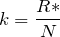# Boltzmann Constant

Boltzmann Constant

Boltzmann Constant

The Boltzmann Constant, k, is a proportional relationship between the kinetic energy of individual particles and temperature. It is defined as the Universal Gas Constant, R*, divided by the Avogadro constant, N.

(1)Where k = Boltzmann constant (J / K), R* = Universal gas constant (J/mol.K), N = Avogadro constant = 6.02214129×10^23 / mol